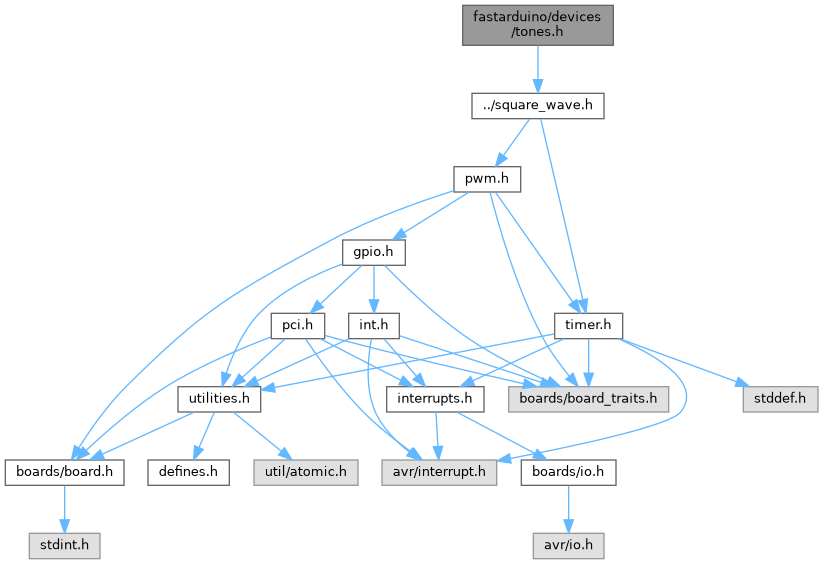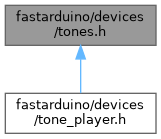FastArduino  v1.7 C++ library to build fast but small Arduino/AVR projects
tones.h File Reference

API to handle tones (simple square waves) generation to a buzzer. More...

`#include "../square_wave.h"`
Include dependency graph for tones.h:This graph shows which files directly or indirectly include this file:Go to the source code of this file.

## Classes

class  devices::audio::ToneGenerator< NTIMER, OUTPUT >
API class for tone generation to a buzzer (or better an amplifier) connected to pin `OUTPUT`. More...

## Namespaces

devices
Defines all API for all external devices supported by FastArduino.

devices::audio
Defines API for audio tones (square waves) generation and simple melodies playing.

## Enumerations

enum  devices::audio::Tone : uint16_t {
USER0 = 0,
USER1,
USER2,
USER3,
USER4,
USER5,
USER6,
USER7,
SILENCE = USER7 + 1,
REST = SILENCE,
C0 = 131,
Cs0 = 139,
Df0 = Cs0,
D0 = 147,
Ds0 = 156,
Ef0 = Ds0,
E0 = 165,
F0 = 175,
Fs0 = 185,
Gf0 = Fs0,
G0 = 196,
Gs0 = 208,
Af0 = Gs0,
A0 = 220,
As0 = 233,
Bf0 = As0,
B0 = 247,
C1 = 262,
Cs1 = 277,
Df1 = Cs1,
D1 = 294,
Ds1 = 311,
Ef1 = Ds1,
E1 = 330,
F1 = 349,
Fs1 = 370,
Gf1 = Fs1,
G1 = 392,
Gs1 = 415,
Af1 = Gs1,
A1 = 440,
As1 = 466,
Bf1 = As1,
B1 = 494,
C2 = 523,
Cs2 = 554,
Df2 = Cs2,
D2 = 587,
Ds2 = 622,
Ef2 = Ds2,
E2 = 659,
F2 = 698,
Fs2 = 740,
Gf2 = Fs2,
G2 = 784,
Gs2 = 831,
Af2 = Gs2,
A2 = 880,
As2 = 932,
Bf2 = As2,
B2 = 988,
C3 = 1046,
Cs3 = 1109,
Df3 = Cs3,
D3 = 1175,
Ds3 = 1245,
Ef3 = Ds3,
E3 = 1319,
F3 = 1397,
Fs3 = 1480,
Gf3 = Fs3,
G3 = 1568,
Gs3 = 1662,
Af3 = Gs3,
A3 = 1760,
As3 = 1865,
Bf3 = As3,
B3 = 1976,
C4 = 2093,
Cs4 = 2217,
Df4 = Cs4,
D4 = 2349,
Ds4 = 2489,
Ef4 = Ds4,
E4 = 2637,
F4 = 2794,
Fs4 = 2960,
Gf4 = Fs4,
G4 = 3136,
Gs4 = 3322,
Af4 = Gs4,
A4 = 3520,
As4 = 3729,
Bf4 = As4,
B4 = 3951
}
This enum defines all possible audio tones that can be generated. More...

## Detailed Description

API to handle tones (simple square waves) generation to a buzzer.

Definition in file tones.h.